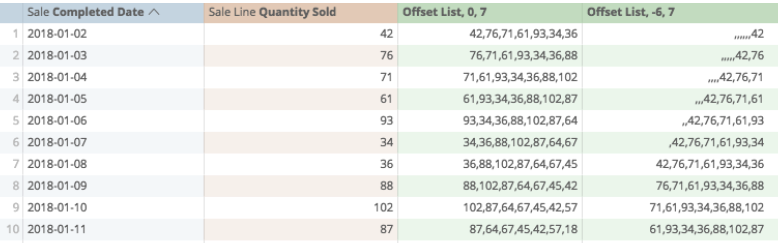# Table functions

Analytics provides many functions that perform different mathematical, logical, and table-based calculations. You can find a more in-depth catalog of available functions on the list of Looker functions and operators page.

To make it easier, we could categorize these in five different ways:

In this article, we'll be looking at six of the Table functions.

## Count

Count returns a count of all non-null values from a range. It requires just one argument — the range being searched: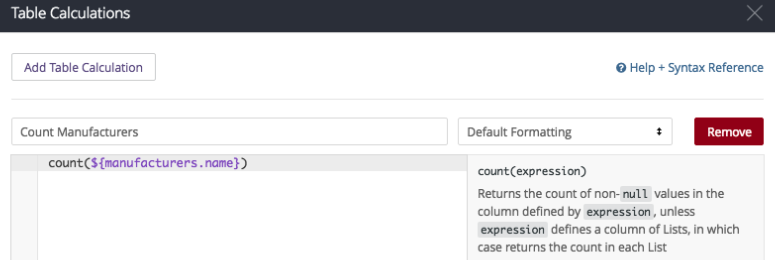So, in this instance, my calculation looks like this:

count(\${manufacturers.name})

If I have prepared the calculation properly: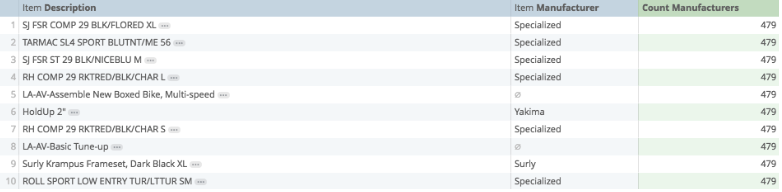## Count distinct

Count distinct counts all unique non-null values from a range and requires one argument — the range being counted: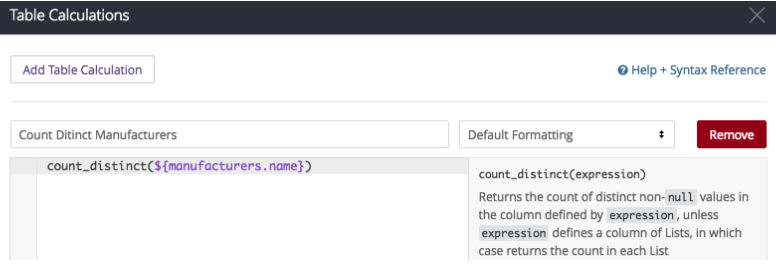In this instance, my calculation looks like this:

count_distinct(\${manufacturers.name})

And if I have prepared my calculation properly, when I save: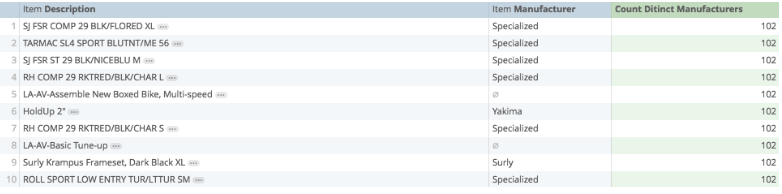## Index

Index is one of the most powerful calculations that returns the content from a specific cell from a defined range. It requires two arguments: the range being looked at and which cell is being returned.

For example, I could ask the calculation to return the third row from the dates: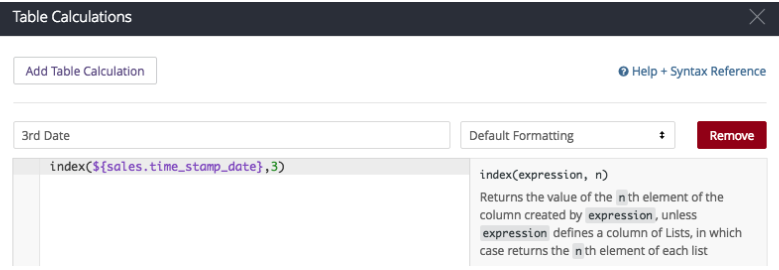in this example, my calculation looks like this:

index(\${sales.time_stamp_date},3)

If I have prepared my calculation properly, when I save: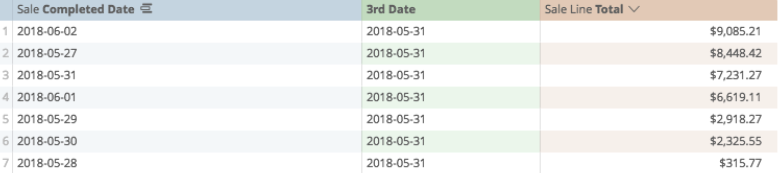## Match

Match is another powerful tool with index that returns the row where content is matched. It requires two arguments: which column is being searched and what content we are looking for.

Say that we are using one calculation to return the largest sale value from the past two weeks, using each row to define the next largest value (sorting from greatest to smallest):

large(\${sale_lines.total_sales_no_tax},row())

Then what we could do is use a match calculation to find which row that largest value was found on: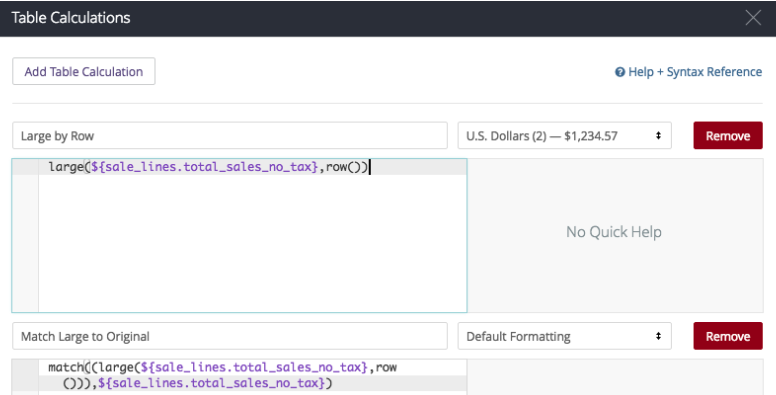So, my calculation looks like this:

match((large(\${sale_lines.total_sales_no_tax},row())),\${sale_lines.total_sales_no_tax})

If I have prepared it properly, when I save: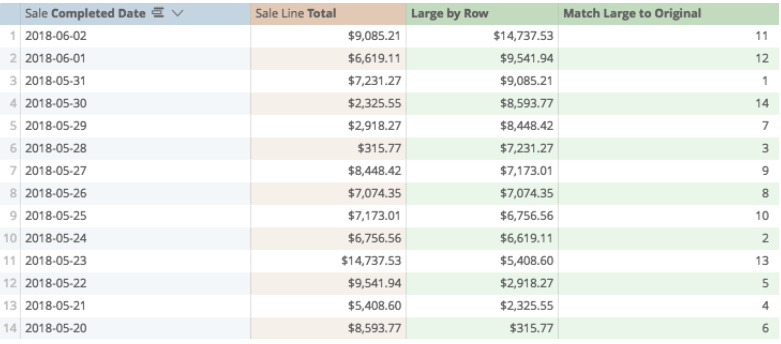Note: While unremarkable on its own, using Match and Index together in compound calculations provides many custom sorting or gathering solutions.

## Offset

Offset returns the value from a range looking up or down a certain number of rows. It requires two arguments: which column is being looked at and how many rows down you wish to look.

In this instance, I'm using Offset positively to look at the row below, and negatively, to look at the row above: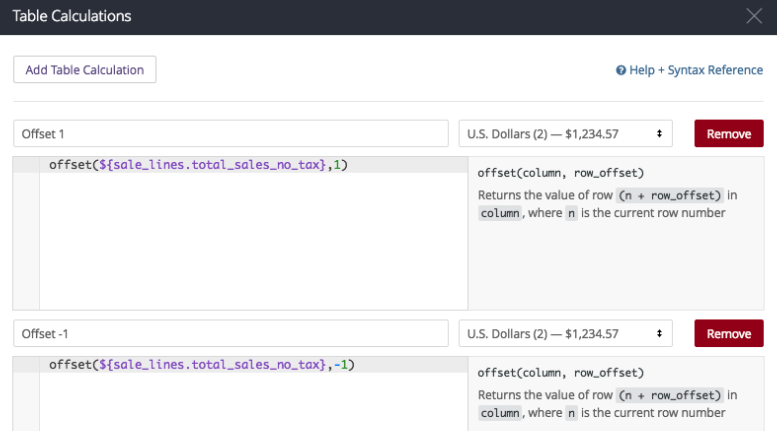My calculations are, respectively:

offset(\${sale_lines.total_sales_no_tax},1)

offset(\${sale_lines.total_sales_no_tax},-1)

If prepared properly, when saved it looks like this: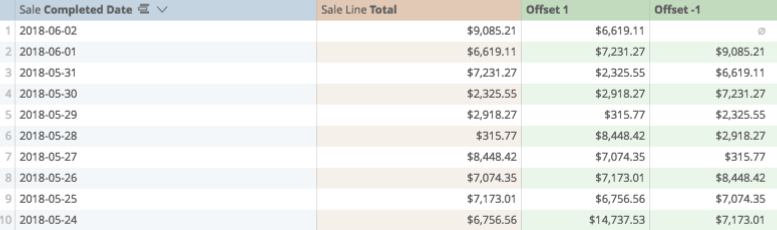## Offset list

Offset list returns a series of numeric or text values from a defined range. It is often used to prepare for summing, such as to illustrate a running weekly total of sales.

It requires three arguments: which range to look at, how many rows down you wish to start at, and how many rows you want to include in the list: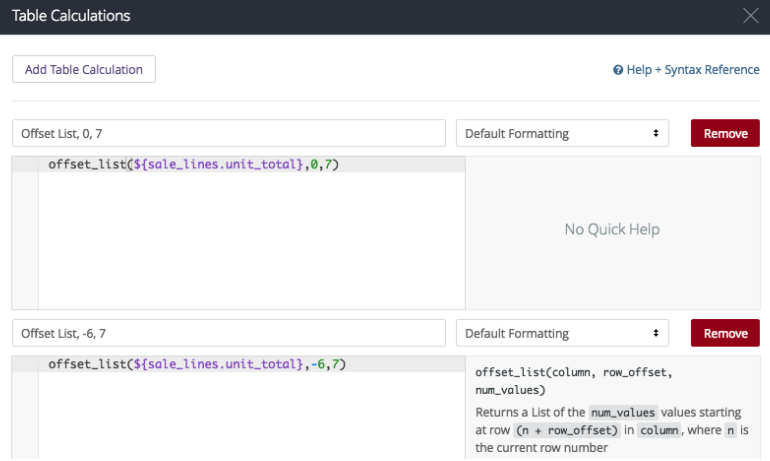In this instance my calculations look like this:

offset_list(\${sale_lines.unit_total},0,7)

Which creates a list from this row up to the seventh row down, and

offset_list(\${sale_lines.unit_total},-6,7)

Which creates a list starting six rows back, and ending on the current row.

If the calculation is properly: